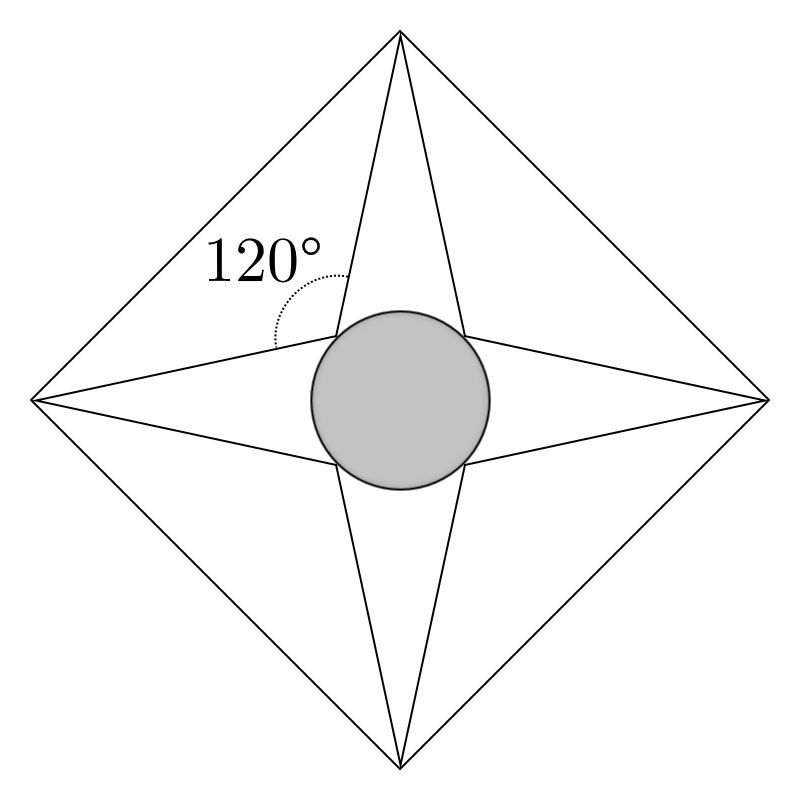# Medals Are For Stars

Geometry Level 2A medal is formed by incsribing a four-pointed star in a diamond of side 1, such that the angle between the points is $120^{\circ}$ as shown.

If the area of the circle inscribed in the star is $\dfrac{a-\sqrt3}{b} \pi$ for integers $a$ and $b$, find $ab$.

×